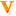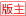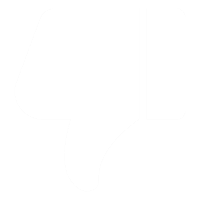·社区中心 社区公告| 政策解答| 学员心声 ·考试专区 中级综合区| 中级会计实务| 中级经济法| 中级财务管理| 初级职称 注会综合区| 会计| 审计| 税法| 经济法| 财管| 风险管理| 综合阶段 税务师| 资产评估师| 高级会计师| 审计师| 经济师 会计学历考试| 国际证书考试| 银行/证券/统计/期货从业 ·互动交流 正保财务软件| 名师专区| 嘉宾访谈| 休闲娱乐| 招聘求职| 会计新人秀 生活与健康| 大学来啦 ·版务专区 南京继续教育| 保定继续教育

# 帖子主题：【计算题】各参数的敏感系数计算发表于：Wed Aug 23 15:10:18 CST 2017

 三千筱夜4497主题帖 11042问答数 论坛等级: 论坛元老 回帖：35883 经验：133337 鲜花：256 金币：11760 离线 用户信息 加为好友 发短消息0点击：600 回复：17    楼主 　　【例题•计算题】甲公司拟加盟乙快餐集团，乙集团对加盟企业采取不从零开始的加盟政策，将已运营2年以上、达到盈亏平衡条件的自营门店整体转让给符合条件的加盟商，加盟经营协议期限15年，加盟时一次性支付450万元加盟费，加盟期内，每年按年营业额的10%向乙集团支付特许经营权使用费和广告费，甲公司预计将于2016年12月31日正式加盟，目前正进行加盟店2017年度的盈亏平衡分析。　　其它相关资料如下：　　(1)餐厅面积400平方米，仓库面积100平方米，每平方米年租金2 400元。　　(2)为扩大营业规模，新增一项固定资产，该资产原值300万元，按直线法计提折旧，折旧年限10年(不考虑残值)。　　(3)快餐每份售价40元，变动制造成本率50%，每年正常销售量15万份。　　假设固定成本、变动成本率保持不变。　　要求：　　(1)计算加盟店年固定成本总额、单位变动成本、盈亏临界点销售额及正常销售量时的安全边际率。　　(2)如果计划目标税前利润达到100万元，计算快餐销售量;假设其他因素不变，如果快餐销售价格上浮5%，以目标税前利润100万元为基数，计算目标税前利润变动的百分比及目标税前利润对单价的敏感系数。　　(3)如果计划目标税前利润达到100万元且快餐销售量达到20万份，计算加盟店可接受的快餐最低销售价格。　　【答案】(1)①固定成本总额=450/15+(400+100)×2400/10000+300/10=180(万元)　　②单位变动成本=40×50%+40×10%=24(元)　　③盈亏零界点的销售额=固定成本/单位边际贡献率，边际贡献率=(40-24)/40=40%；所以盈亏零界点的销售额=180/40%=450(万元)　　④正常销售量的安全边际率=1-盈亏临界点的销售量/正常销售量=1-(450/40)/15=25%　　(2)①(单价-单位变动成本)×销售量-固定成本=利润；则(40-24)×销售量-180=100　　销售量=17.5(万件)　　②单价上升5%，　　利润=40×(1+5%)×(1-50%-10%)×17.5-180=114(万元)　　利润变化率=(114-100)/100=14%　　利润对单价的敏感系数=14%/5%=2.8　　(3)单价×(1-50%-10%)×20-180=100；可以接受的最低单价=35(元)。 悬赏金额: 10 金币 状态： 未解决 引用 回复 收藏 求助 送鲜花 举报 相关热帖 楼主其他文章 · 看跌期权价值的计算 · 会导致应收账款周转天数减少的条件 · 净现值和现值指数，多选题 · 增发新股 · 可能导致企业权益乘数降低的经济业务 · 关于资产负债率、权益乘数和产权比率之间关系 · 【我猜这些题需要加强化】 总债务存量比率测试 · 外部融资额
 三千筱夜4497主题帖 11042问答数 论坛等级: 论坛元老 回帖：35883 经验：133337 鲜花：256 金币：11760 离线 用户信息01楼   发表于：2017-08-23 15:10:45 只看该作者@四叶草2014 @xuan_hh @猴子娅 @benermaru @cc向前冲 @momoi @xingfurensheng1987 @普雅花开 @蛋炒蛋 @2447417495 @重生 @15999968495 @财艺新文 @sherrybaby @随风思念 @古城and狐狸 @燕格格chy @ouyangde @jiangguofuma @xiaojane0810
 357主题帖 8914问答数 论坛等级: 终极会员 回帖：14200 经验：34251 鲜花：1129 金币：80248 离线 用户信息02楼   发表于：2017-08-23 23:54:44 只看该作者 (1)计算加盟店年固定成本总额、单位变动成本、盈亏临界点销售额及正常销售量时的安全边际率。加盟店年固定成本=450/15+0.24*(400+100)+300/10=180(万元）变动成本率=50%+10%=60% 单位变动成本=40*60%=24（元）盈亏临界点销售额=180/[(40-24）/40]=450（万元）安全边际率=（40*15-450）/40*15=25%. 三千筱夜 于 Tue Aug 29 10:51:27 CST 2017 回复： 【答案】(1)①固定成本总额=450/15+(400+100)×2400/10000+300/10=180(万元)　　②单位变动成本=40×50%+40×10%=24(元)　　③盈亏零界点的销售额=固定成本/单位边际贡献率，边际贡献率=(40-24)/40=40%；所以盈亏零界点的销售额=180/40%=450(万元)　　④正常销售量的安全边际率=1-盈亏临界点的销售量/正常销售量=1-(450/40)/15=25%　　(2)①(单价-单位变动成本)×销售量-固定成本=利润；则(40-24)×销售量-180=100　　销售量=17.5(万件)　　②单价上升5%，　　利润=40×(1+5%)×(1-50%-10%)×17.5-180=114(万元)　　利润变化率=(114-100)/100=14%　　利润对单价的敏感系数=14%/5%=2.8　　(3)单价×(1-50%-10%)×20-180=100；可以接受的最低单价=35(元)。
 357主题帖 8914问答数 论坛等级: 终极会员 回帖：14200 经验：34251 鲜花：1129 金币：80248 离线 用户信息03楼   发表于：2017-08-23 23:56:36 只看该作者 (2)如果计划目标税前利润达到100万元，计算快餐销售量;假设其他因素不变，如果快餐销售价格上浮5%，以目标税前利润100万元为基数，计算目标税前利润变动的百分比及目标税前利润对单价的敏感系数。目标税前利润达到100万元的销售量=（180+100）/[40*(1-60%)]=17.5(万份）目标税前利润变动的百分比= 17.5*[40*(1+5%)*(l-60%)-40*(1-60%)]/100=14% 目标税前利润对单价的敏感系数=14%/5%=2.8 三千筱夜 于 Tue Aug 29 10:51:33 CST 2017 回复： 【答案】(1)①固定成本总额=450/15+(400+100)×2400/10000+300/10=180(万元) 　　②单位变动成本=40×50%+40×10%=24(元)　　③盈亏零界点的销售额=固定成本/单位边际贡献率，边际贡献率=(40-24)/40=40%；所以盈亏零界点的销售额=180/40%=450(万元)　　④正常销售量的安全边际率=1-盈亏临界点的销售量/正常销售量=1-(450/40)/15=25%　　(2)①(单价-单位变动成本)×销售量-固定成本=利润；则(40-24)×销售量-180=100　　销售量=17.5(万件)　　②单价上升5%，　　利润=40×(1+5%)×(1-50%-10%)×17.5-180=114(万元)　　利润变化率=(114-100)/100=14%　　利润对单价的敏感系数=14%/5%=2.8　　(3)单价×(1-50%-10%)×20-180=100；可以接受的最低单价=35(元)。
 357主题帖 8914问答数 论坛等级: 终极会员 回帖：14200 经验：34251 鲜花：1129 金币：80248 离线 用户信息04楼   发表于：2017-08-23 23:57:44 只看该作者 (3)如果计划目标税前利润达到100万元且快餐销售量达到20万份，计算加盟店可接受的快餐最低销售价格。可接受的最低销售价格=（180+100）/[20*(1-60%)]=35 (元） 三千筱夜 于 Tue Aug 29 10:51:39 CST 2017 回复： 【答案】(1)①固定成本总额=450/15+(400+100)×2400/10000+300/10=180(万元) 　　②单位变动成本=40×50%+40×10%=24(元)　　③盈亏零界点的销售额=固定成本/单位边际贡献率，边际贡献率=(40-24)/40=40%；所以盈亏零界点的销售额=180/40%=450(万元)　　④正常销售量的安全边际率=1-盈亏临界点的销售量/正常销售量=1-(450/40)/15=25%　　(2)①(单价-单位变动成本)×销售量-固定成本=利润；则(40-24)×销售量-180=100　　销售量=17.5(万件)　　②单价上升5%，　　利润=40×(1+5%)×(1-50%-10%)×17.5-180=114(万元)　　利润变化率=(114-100)/100=14%　　利润对单价的敏感系数=14%/5%=2.8　　(3)单价×(1-50%-10%)×20-180=100；可以接受的最低单价=35(元)。
 xi1972336主题帖 8836问答数 论坛等级: 终极会员 回帖：14484 经验：33669 鲜花：1005 金币：43412 离线 用户信息05楼   发表于：2017-08-24 07:39:58 只看该作者三千筱夜 于 Tue Aug 29 10:51:47 CST 2017 回复： 【答案】(1)①固定成本总额=450/15+(400+100)×2400/10000+300/10=180(万元) 　　②单位变动成本=40×50%+40×10%=24(元)　　③盈亏零界点的销售额=固定成本/单位边际贡献率，边际贡献率=(40-24)/40=40%；所以盈亏零界点的销售额=180/40%=450(万元)　　④正常销售量的安全边际率=1-盈亏临界点的销售量/正常销售量=1-(450/40)/15=25%　　(2)①(单价-单位变动成本)×销售量-固定成本=利润；则(40-24)×销售量-180=100　　销售量=17.5(万件)　　②单价上升5%，　　利润=40×(1+5%)×(1-50%-10%)×17.5-180=114(万元)　　利润变化率=(114-100)/100=14%　　利润对单价的敏感系数=14%/5%=2.8　　(3)单价×(1-50%-10%)×20-180=100；可以接受的最低单价=35(元)。 有志者事竟成
 394主题帖 1631问答数 论坛等级: 钻石会员 回帖：2948 经验：10368 鲜花：30 金币：9917 离线 用户信息06楼   发表于：2017-08-26 09:45:34 只看该作者 谢谢分享 三千筱夜 于 Tue Aug 29 10:51:53 CST 2017 回复： 【答案】(1)①固定成本总额=450/15+(400+100)×2400/10000+300/10=180(万元) 　　②单位变动成本=40×50%+40×10%=24(元)　　③盈亏零界点的销售额=固定成本/单位边际贡献率，边际贡献率=(40-24)/40=40%；所以盈亏零界点的销售额=180/40%=450(万元)　　④正常销售量的安全边际率=1-盈亏临界点的销售量/正常销售量=1-(450/40)/15=25%　　(2)①(单价-单位变动成本)×销售量-固定成本=利润；则(40-24)×销售量-180=100　　销售量=17.5(万件)　　②单价上升5%，　　利润=40×(1+5%)×(1-50%-10%)×17.5-180=114(万元)　　利润变化率=(114-100)/100=14%　　利润对单价的敏感系数=14%/5%=2.8　　(3)单价×(1-50%-10%)×20-180=100；可以接受的最低单价=35(元)。
 xi1972336主题帖 8836问答数 论坛等级: 终极会员 回帖：14484 经验：33669 鲜花：1005 金币：43412 离线 用户信息07楼   发表于：2017-08-28 07:35:52 只看该作者三千筱夜 于 Tue Aug 29 10:51:59 CST 2017 回复： 【答案】(1)①固定成本总额=450/15+(400+100)×2400/10000+300/10=180(万元) 　　②单位变动成本=40×50%+40×10%=24(元)　　③盈亏零界点的销售额=固定成本/单位边际贡献率，边际贡献率=(40-24)/40=40%；所以盈亏零界点的销售额=180/40%=450(万元)　　④正常销售量的安全边际率=1-盈亏临界点的销售量/正常销售量=1-(450/40)/15=25%　　(2)①(单价-单位变动成本)×销售量-固定成本=利润；则(40-24)×销售量-180=100　　销售量=17.5(万件)　　②单价上升5%，　　利润=40×(1+5%)×(1-50%-10%)×17.5-180=114(万元)　　利润变化率=(114-100)/100=14%　　利润对单价的敏感系数=14%/5%=2.8　　(3)单价×(1-50%-10%)×20-180=100；可以接受的最低单价=35(元)。 有志者事竟成
 wrfwrf20121777主题帖 7466问答数 论坛等级: 论坛元老 回帖：18307 经验：59222 鲜花：748 金币：116694 离线 用户信息08楼   发表于：2017-08-29 14:44:12 只看该作者 练练手 三千筱夜 于 Wed Aug 30 17:02:08 CST 2017 回复： 【答案】(1)①固定成本总额=450/15+(400+100)×2400/10000+300/10=180(万元) 　　②单位变动成本=40×50%+40×10%=24(元)　　③盈亏零界点的销售额=固定成本/单位边际贡献率，边际贡献率=(40-24)/40=40%；所以盈亏零界点的销售额=180/40%=450(万元)　　④正常销售量的安全边际率=1-盈亏临界点的销售量/正常销售量=1-(450/40)/15=25%　　(2)①(单价-单位变动成本)×销售量-固定成本=利润；则(40-24)×销售量-180=100　　销售量=17.5(万件)　　②单价上升5%，　　利润=40×(1+5%)×(1-50%-10%)×17.5-180=114(万元)　　利润变化率=(114-100)/100=14%　　利润对单价的敏感系数=14%/5%=2.8　　(3)单价×(1-50%-10%)×20-180=100；可以接受的最低单价=35(元)。
 wrfwrf20121777主题帖 7466问答数 论坛等级: 论坛元老 回帖：18307 经验：59222 鲜花：748 金币：116694 离线 用户信息09楼   发表于：2017-08-31 14:45:17 只看该作者 练练手 三千筱夜 于 Fri Sep 01 15:09:12 CST 2017 回复：# 【计算题】各参数的敏感系数计算您尚未登录,发表回复前请先登录，或者注册
 Ctrl+Enter直接发表